“The great wall is made by blocks”

# 二进制加法

## 半加器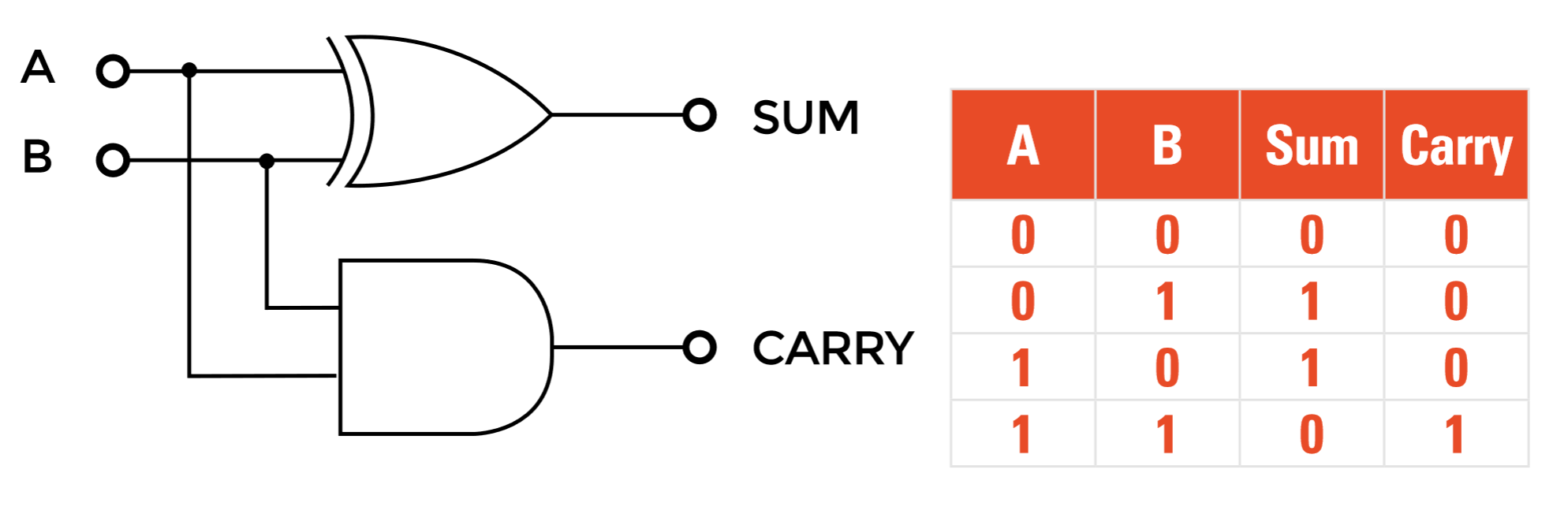## 全加器

c b a sum(out) carry(out)
0 0 0 0 0
0 0 1 1 0
0 1 0 1 0
0 1 1 0 1
1 0 0 1 0
1 0 1 0 1
1 1 0 0 1
1 1 1 1 1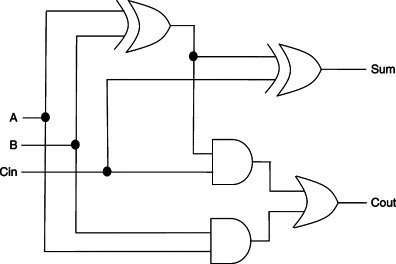## 多位加法器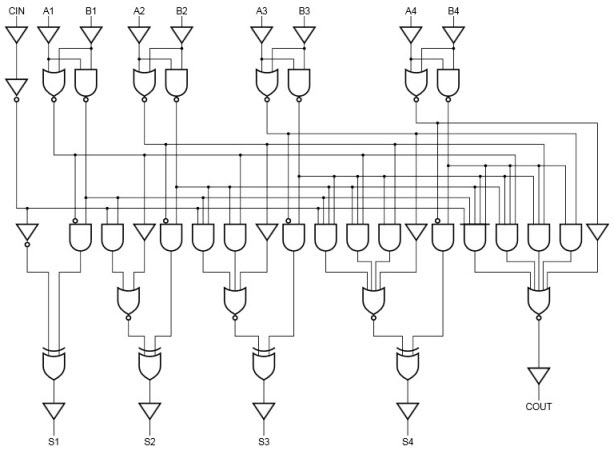# 负数

$\textrm{Input}: x$

$\textrm{Output}: -x$

# 算数逻辑单元（ALU）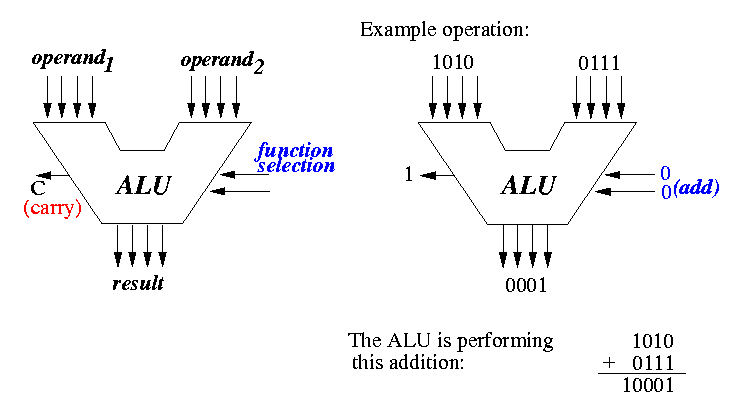ALU：输入两个操作数和待执行的函数（算数或逻辑运算），给出指定结果，对于本课程的Hack ALU，其结构如下所示：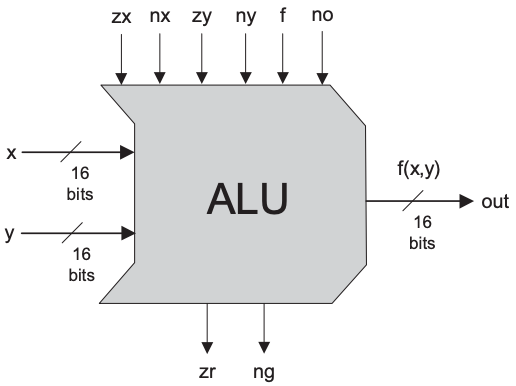0 x x & y -x
1 y x \ y -y
-1 !x x+1
!y y+1
x-1
y-1
x+y
x-y
y-x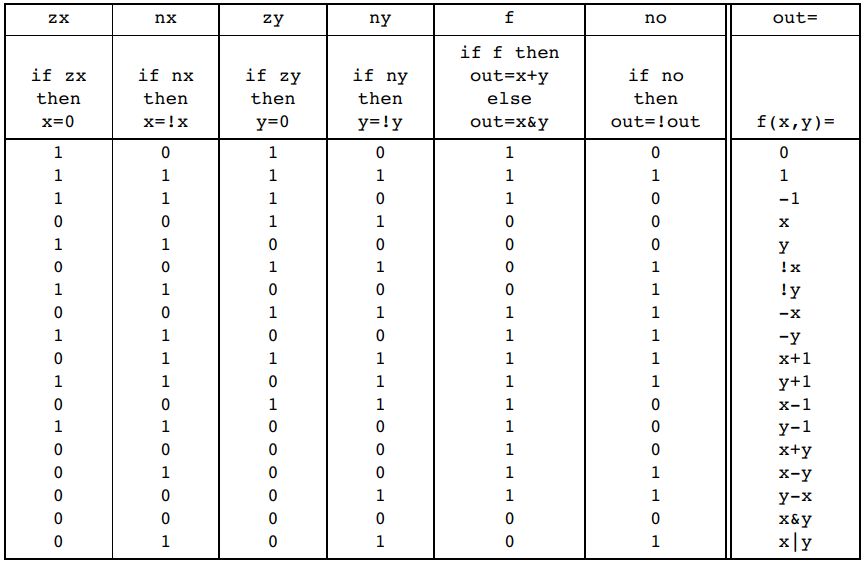• 计算1：为了计算1，我们可以对齐按位取反，得到b’1110，而b’1110为$-2$，即$-1+(-1)$，故只需要将$x$与$y$归零并按位取反即可
• 计算$-x$：$-x=\overline x+1$，故$-x-1=\overline x$，两边同时取反，可得$\overline{-x-1}=x$，令$x=-x$，即可得到$\overline{x-1}=-x$。
• 计算$x-y$：

0%